## Algebra

Showing posts with label distributive property. Show all posts
Showing posts with label distributive property. Show all posts

### Multiplying Polynomials

The distributive property and the product rule for exponents are the keys to multiplication of polynomials.

Remember the product rule,It says that when we multiply two numbers with the same base we must add the exponents.

Monomial x PolynomialMultiply.A common mistake is to distribute when working with multiplication. The distributive property only applies when addition or subtraction separates the terms.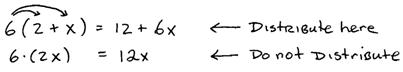Binomial x Polynomial: Use the distributive property to multiply polynomials by binomials.We can save a step by distributing each term in the binomial individually. Sometimes this technique is referred to as the FOIL method. (FOIL – Multiply the First, Outer, Inner and then Last terms together.)

Multiply.When combining like terms, be sure that the variable parts are exactly the same. Go slow and work in an organized fashion because it is easy to make an error when many terms are involved.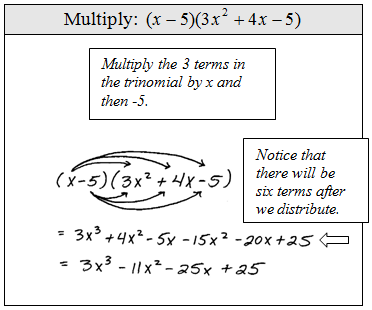It is good practice to recheck your distributive step.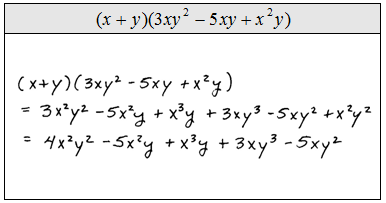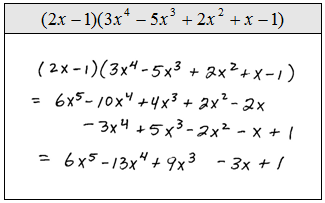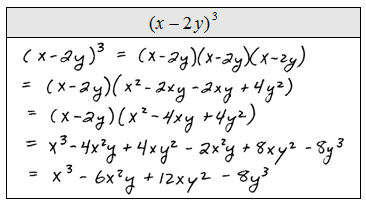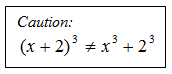Trinomial x Polynomial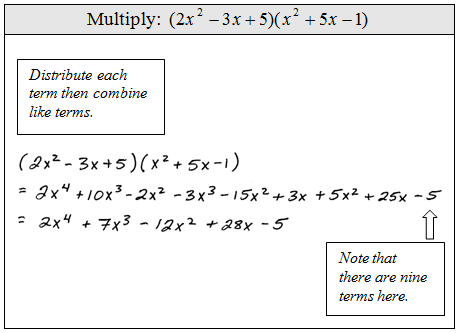The following problem is one that we will have to be able to do before moving on to the next algebra course. These are tedious, time consuming, and often worked incorrectly. Use caution, take your time and work slowly.Function notation for multiplication looks likeGiven the functions f and g find (f g)(x).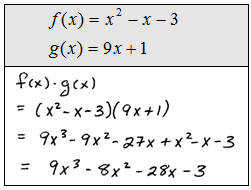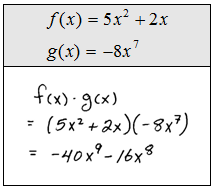The following special products will simplify things if we memorize them.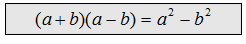This special product is often called difference of squares. Notice that the middle terms cancel because one term will always be positive and the other will be negative.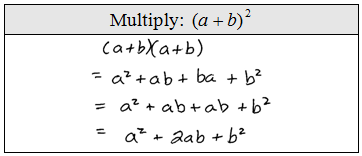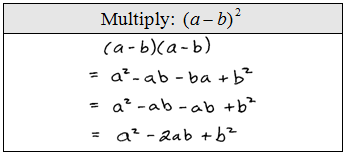We may use these formulas as templates when multiplying binomials. It is a good idea to memorize them.

Multiply.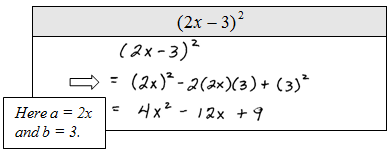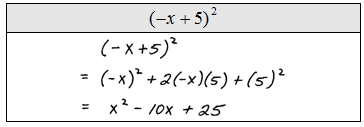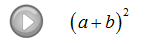### Simplifying Algebraic Expressions

The properties of real numbers are very important in our study of Algebra. These properties can be applied in Algebra because a variable is simply a letter that represents a real number. The distributive property is one that we apply often when simplifying algebraic expressions. Given real numbers a, b, c:
a(b + c) = ab + ac
When multiplying an expression within parentheses you must multiply everything inside by the number or variable that you are distributing.Introduction to the Distributive Property

Simplify.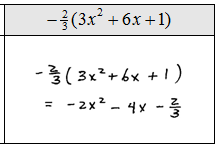When simplifying, we will often have to combine like terms after we distribute.  This step is consistent with the order of operations, multiplication before addition.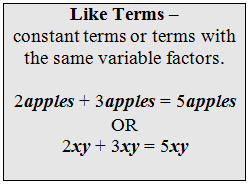Combining Like Terms

Simplify.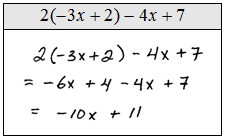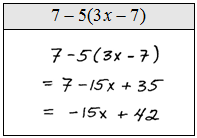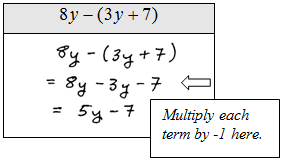Simplifying Algebraic Expressions

To combine like terms, the variable parts have to be exactly the same.  But before combining like terms, generally, we will first distribute if necessary.  When distributing negative numbers notice that the operations change.

Simplify.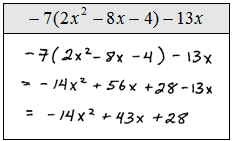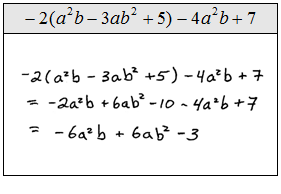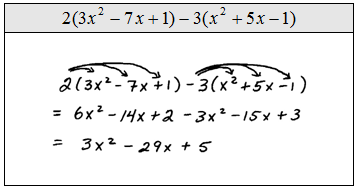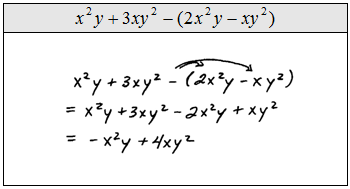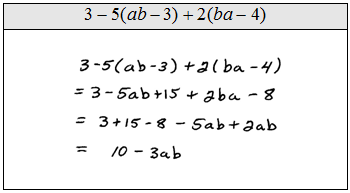Profit Word Problem: Profit is equal to revenues less cost of production.  If the revenue R can be represented byand the cost C can be represented bywhere x represents the number of units produced, find an equation that represents the profit.Subtracting Variable Expressions: What is the difference between 3x − 4 and −2x + 5?